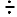Name:    2nd Grade Math Basic Test 1

Multiple Choice
Identify the choice that best completes the statement or answers the question.

1.

Algebraic Concepts – Solving Equations & Inequalities – RIT 161 – 170
7 + ___ = 9
 a. 3 d. 1 b. 2 e. 5 c. 4

2.

Ten cars were in a race.  Two cars did not finish.  How would you find the number of cars that did finish?
 a. add (+) d. divide () b. subtract (-) e. none of the above c. multiply (x)

3.

Algebraic Concepts – Properties – RIT 171 – 180
2 x 3 = 3 x ____
 a. 6 d. 2 b. 5 e. 9 c. 3

4.

Algebraic Concepts – Solving Equations & Inequalities – RIT 171 – 180
Find the missing factor.
3 x ___  = 15
 a. 4 d. 12 b. 5 e. 7 c. 6

5.

6 + 3 - 4 = ____
 a. 13 d. 5 b. 7 e. 10 c. 9

6.

Computation – Whole Numbers – RIT < 150
3 + 1 = 4
1 + 3 = 4
4 – 1 = 3
Which fact is missing?
 a. 4 – 3 = 1 c. 3 + 4 = 7 b. 4 + 3 = 7 d. 3 + 2 = 5

7.

5
-2
 a. 5 d. 7 b. 3 e. 6 c. 2

8.

****  +  *** = _____
 a. 5 d. 4 b. 3 e. 6 c. 7

9.

3
1
+4
 a. 7 d. 8 b. 5 e. 4 c. 9

10.

125
+43
 a. 179 d. 199 b. 168 e. 158 c. 62

11.

12
-5
 a. 5 d. 7 b. 8 e. 17 c. 6

12.

*    *         *    *          *    *
*              *                 *
3 x 3 =
 a. 9 d. 3 b. 6 e. 8 c. 12

13.

Computation – Decimals – RIT 161 – 170

\$5.25
-  3.14
 a. \$1.21 d. \$2.39 b. \$2.11 e. \$4.31 c. \$8.39

14.

Computation – Whole Numbers – RIT 161 – 170
#  #  #               #  #
#  #  #        -     #   #     =  ______
 a. 10 d. 4 b. 3 e. 5 c. 2

15.

63
+34
 a. 37 d. 98 b. 97 e. 31 c. 71